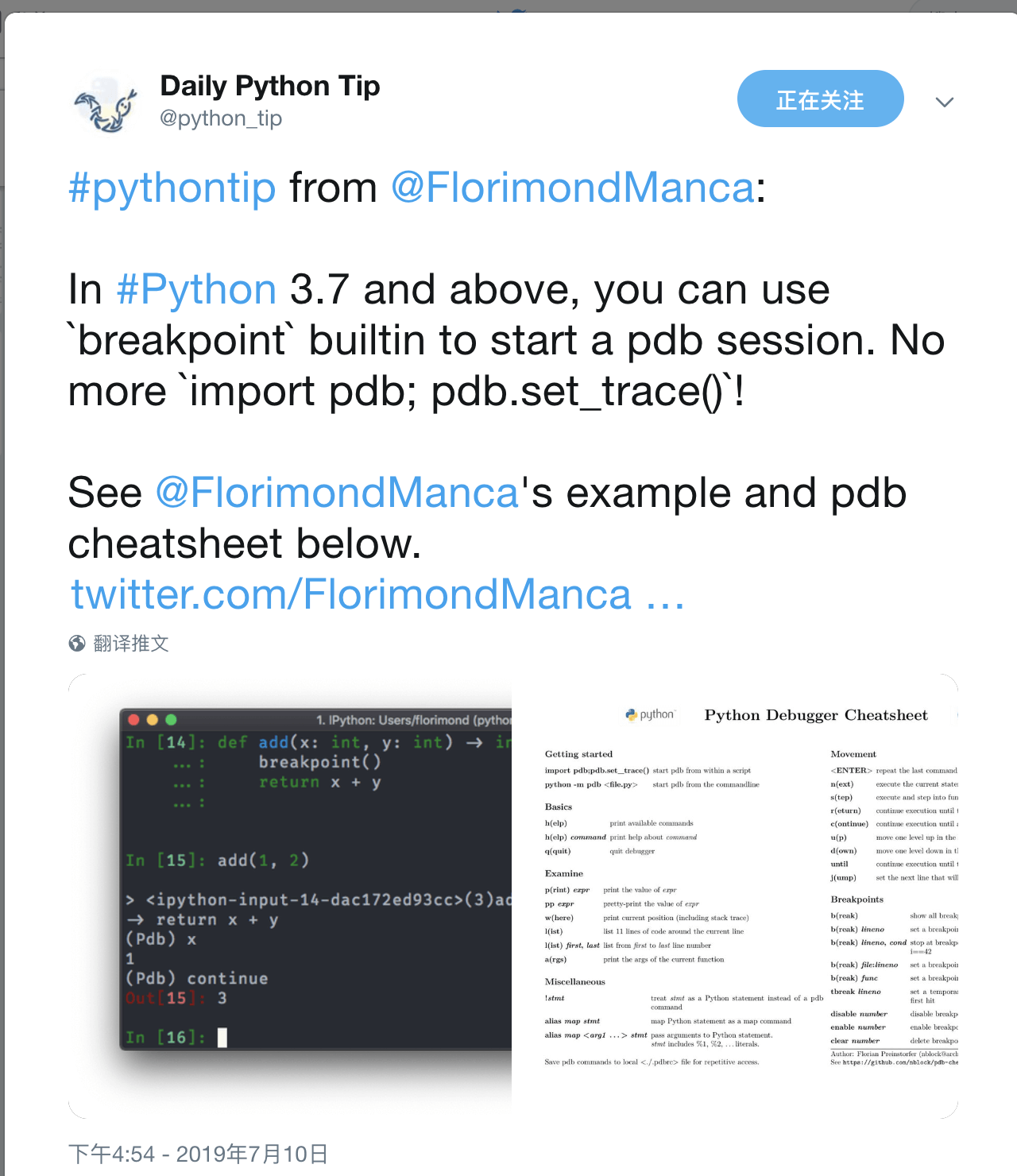ARTS 第十四周
Toc
ARTS Week-14## Algorithm

131. 分割回文串

给定一个字符串 s，将 s 分割成一些子串，使每个子串都是回文串。

[
["aa","b"],
["a","a","b"]
]


class Solution:
ans = None
length = None
s = None
def partition(self, s: str) -> List[List[str]]:
self.ans = []

if not s:
return self.ans

self.s = s
self.length = len(s)

self._partition()
return self.ans

def _partition(self, start=0, res=None):
if res is None:
res = []

if res is None:
res = []

if start >= self.length:
self.ans.append(res)
return

for end in range(start + 1, self.length + 1):
sub = self.s[start: end]
if sub == sub[::-1]:
self._partition(end, res + [sub])


## Review

《This computer changed the world—and you’ve never heard about it》

## Tip## Share